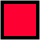CDAM: Computational, Discrete and Applicable Mathematics@LSECDAM Research Report, LSE-CDAM-2007-33

December 2007

A Sublinear-Time Approximation Scheme for Bin Packing

Tugkan Batu, Petra Berenbrink, and Christian Sohler

The bin packing problem is defined as follows: given a set of n items with sizes 01,w2,..., wn≤ 1, find a packing of these items into minimum number of unit-size bins possible.

We present a sublinear-time asymptotic approximation scheme for the bin packing problem; that is, for any ε>0, we present an algorithm Aε that has sampling access to the input instance and outputs a value k such that Copt≤ k≤ (1+ε) Copt+1, where Copt is the cost of an optimal solution. It is clear that uniform sampling by itself will not allow a sublinear-time algorithm in this setting; a small number of items might constitute most of the total weight and uniform samples will not hit them. In this work we use weighted samples, where item i is sampled with probability proportional to its weight: that is, with probability wi/∑i wi. In the presence of weighted samples, the approximation algorithm runs in O(n1/2 poly(log(n)/ε)) + g(1/ε) time, where g(x) is an exponential function of x. When both weighted and uniform sampling are allowed, O(n1/3 poly(log(n)/ε)) + g(1/ε) time suffices. In addition to an approximate value to Copt, our algorithm can also output a constant-size ``template'' of a packing that can later be used to find a near-optimal packing in linear time.

A PDF file (196 kB) with the full contents of this report can be downloaded by clicking here.

Alternatively, if you would like to get a free hard copy of this report, please send the number of this report, LSE-CDAM-2007-33, together with your name and postal address to:CDAM Research Reports Series Centre for Discrete and Applicable Mathematics London School of Economics Houghton Street London WC2A 2AE, U.K.Phone: +44(0)-20-7955 7494. Fax: +44(0)-20-7955 6877. Email: info@maths.lse.ac.uk